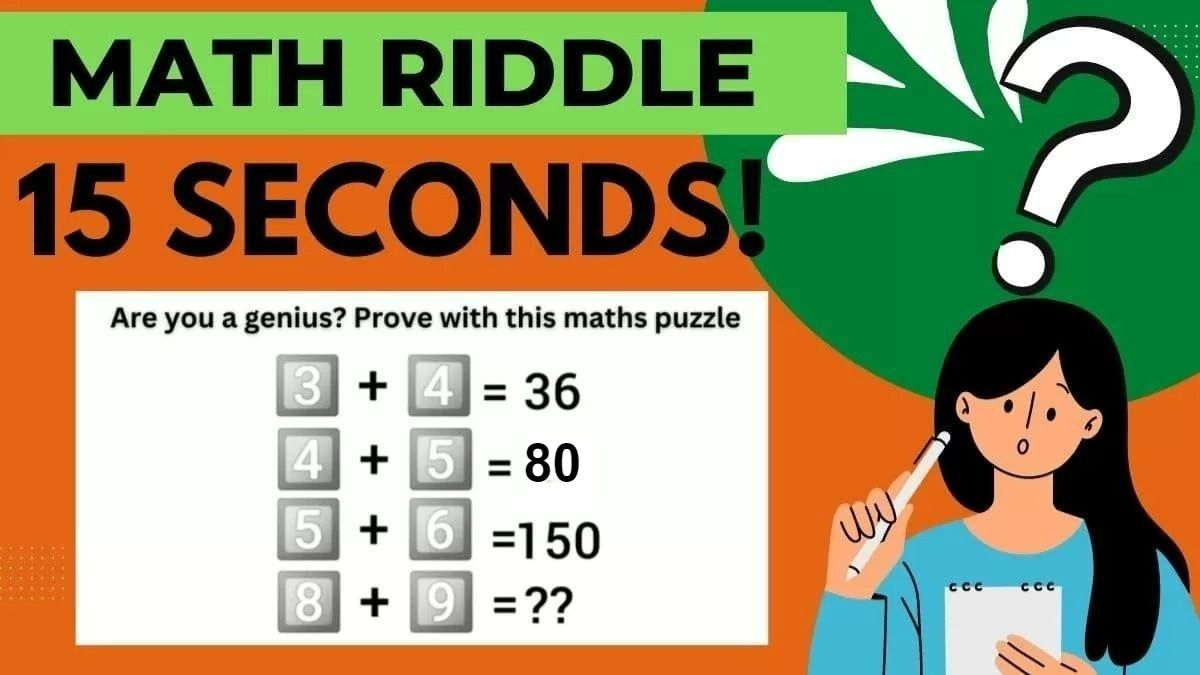# Math Riddles: Are You A Genius? Can You Solve This Key Math Puzzle In 15 Seconds? Test Your IQ Here!

Math Riddle Challenge: Feeling sleepy? We have the perfect math riddle challenge for you to get your brain active and running! Think you are a genius? Prove with this maths puzzle is a type of puzzle that is designed to challenge and stimulate the brain.Math Riddles: Are You A Genius? Can You Solve This Math Puzzle In 15 Seconds? Test Your IQ Here!

Math Riddle Challenge: Feeling sleepy? We have the perfect math riddle challenge for you to get your brain active and running! Think you are a genius? Prove with this maths puzzle is a type of puzzle that is designed to challenge and stimulate the brain. They require a certain level of critical thinking, problem-solving, and logical reasoning skills in order to solve. Can you do it?

What are Math riddles?

Math Riddles are puzzle games where players try to figure out a solution to a problem. As fun as they are, the practice also challenges your brain. They are a great way to boost mental capacity and lateral thinking skills, maintain memory strength, and delight you! It often requires thinking unconventionally with given constraints in mind; sometimes, it involves lateral thinking.

Let’s find out if all of this is true or not! Are you ready?

Here we go!

## Math Riddle Challenge

Look at the picture posted below. You will find a math puzzle that only those with a high IQ will be able to answer. Carefully check this math puzzle and find the missing number.

Is it easy?

Were you able to solve this tricky math puzzle?

If you were able to solve it, let's make it a challenge.

Set a timer to 15 seconds.

Find out how fast you can solve this math puzzle. If you can solve this puzzle in less time, then that's even better.

Now, go ahead and bring out your mathematical brain to solve this tricky puzzle. Your time starts now.

All the best!

Tick…

Tock…

Tick..

Time’s Up!

 Math fact! The word “hundred” comes from the old Norse term, “hundrath”, which actually means 120 and not 100.

Have you solved the brain teaser yet? We are going to reveal the answers now. Let’s solve it together.

## Solution

To solve a mathematical puzzle, observe the puzzle and try to solve it to find the solution to the problem. If you cannot find the solution, we have an answer for you. If you are still working to figure it out, we have the solution to this mathematical puzzle. This puzzle will allow you to see how keen you are and how reasonable an observer you are. If you still need help, the picture below will help you understand the solution.

Since you have gone through the image, there is a chance that you have some idea about the puzzle we have given. If you are still going through the puzzle, no worries; take your time, and there is a chance for you to find the solution for the image. So, make your brain get some ideas about the puzzle given and use your mathematics skills to their full extent to solve this problem.

## So the answer is 576.

Good Work!

TRY THESE TOO!

Math Riddles: Can you Solve this Math Sequence Puzzle In 15 Seconds? Test your IQ here!

Math Riddles: Can you Solve a Math Table Puzzle In 15 Seconds? Test your IQ here!

Math Riddles: Can You Solve This Heart Puzzle In 30 Seconds?

Get the latest General Knowledge and Current Affairs from all over India and world for all competitive exams.
खेलें हर किस्म के रोमांच से भरपूर गेम्स सिर्फ़ जागरण प्ले पर